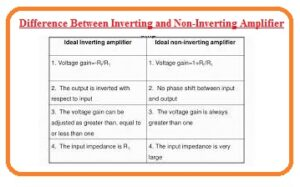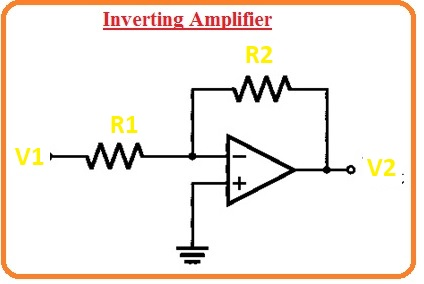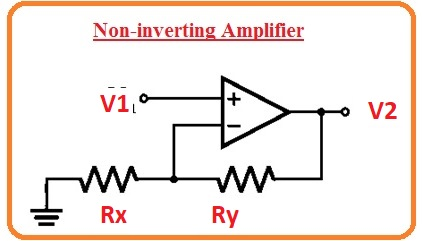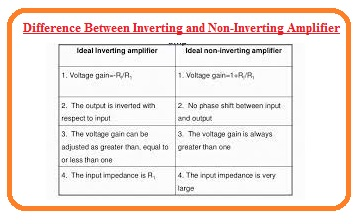Hello, friends welcome to the new post. In this post, we will have a detailed look at Difference Between Inverting and Non-Inverting Amplifier. the operational amplifiers ic circuit used to do amplification of voltage. This circuit is created through the use of resistances and capacitors. The main features offered by this device are the gain of an infinity open loop, its infinite resistance, output resistance is .

It consists of 2 terminals first two are inputs and third one is output. Inputs terminal are inverting and non inverting. Generally, practical implementation of the meths operation are done by the circuit. Here we cover the basics of these circuits with the details so let’s get started.

## Difference Between Inverting and Non-Inverting Amplifier

Introduction to Inverting Amplifier

• This category of the amplifier provides the output at an angle of one-eight degrees than input. IF a positive value of supply is provided to this circuitry output of the circuitry will be negative.
• Here you can see the diagram of this circuitry.
• If we consider this circuitry is ideal then there is short circuitry among the input points of the operational amplifier.
• That caused the voltage equal at 2 points of inputs terminals.
• After application of KCL to the above circuitry we have

(0-Vq)/R1 + (0−V2) /( R2) = 0

• The voltage gain of this circuitry is given here.

-R2/R1Introduction to Non-inverting Amplifier

• In this circuitry configuration, there is not out of phase behavior for output related to the input. if the voltage applied is positive then its output will also be positive.
• The output is noninverting. Here we can see the circuit of this amplifier.
• In the case of an ideal operational amplifier, there is a similar value of the voltage at 2 points input terminals.
• After application of KCL we has.

(V1−V2)/R2 + (V2−0)/(Rx) = 0

• Its gain value is

AV=1+Ry/Rx### Comparison Between Inverting and Non-Inverting AmplifierWhat is Inverting Amplifier

• The output of this amplifier is out of phase the signal given at input.
• There is one-eighty degrees of phase difference between input and output.
• Input is given at non-inverting terminal or negative of circuitry.
• Its polarity is negative than another amplifier.
• It’s gaining the ratio of resistance at output and input.
• Its polarity value is negative.

What is a Non-Inverting Amplifier

• The output of this amplifier is in phase to the input given at this circuitry.
• There is zero phase difference between input and output.
• Its input is given at the positive terminal of circuitry.
• Its output has noninverted behavior than inverting amplifier.
• Its gain polarity is positive.
• Gin is the sum of one and the ratio of resistances.

That is a detailed post about the Difference Between Inverting and Non-Inverting Amplifier if you has any further query ask in the comments. Thanks for reading have a nice day.